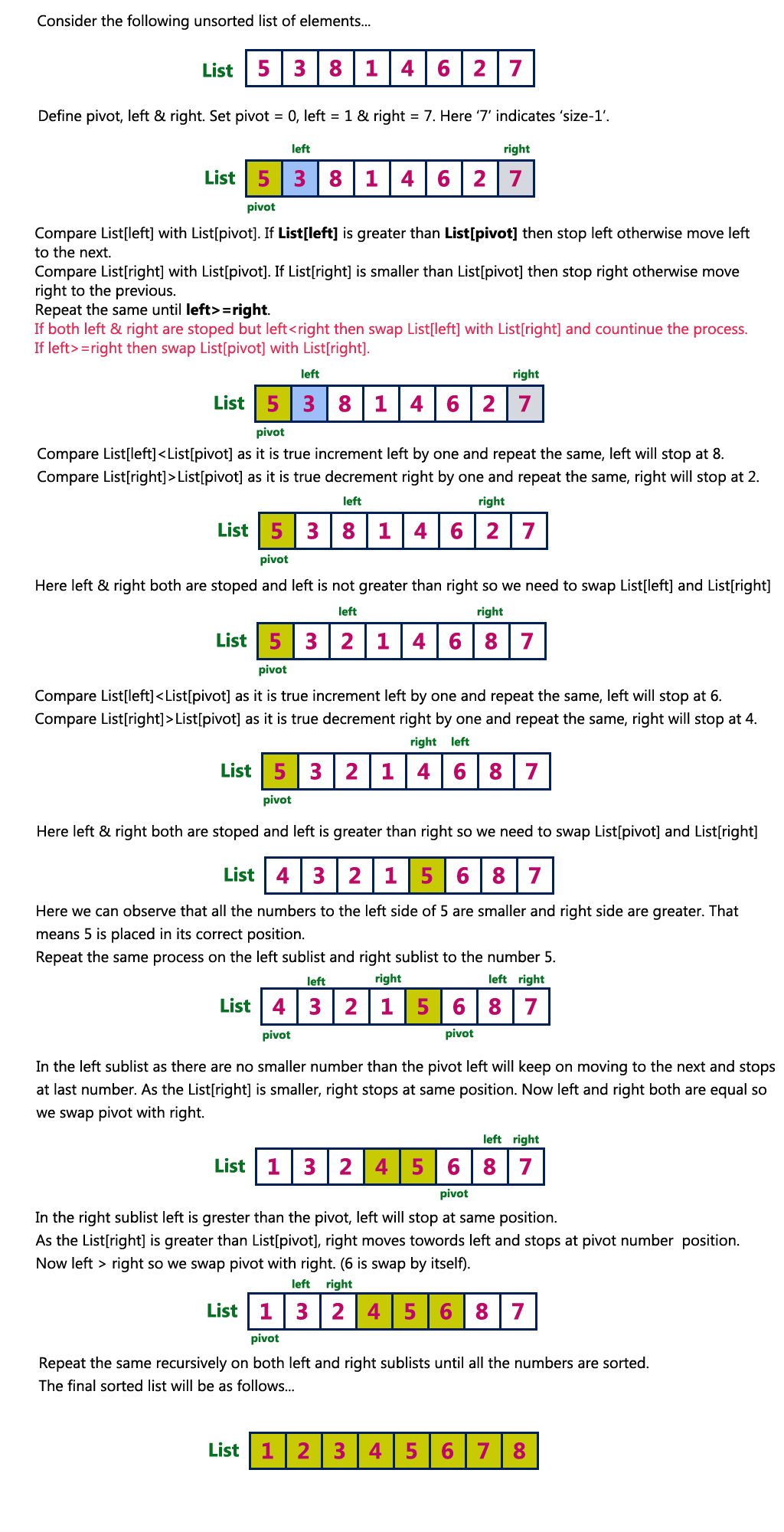The perfect place for easy learning...

×

# Quick Sort Algorithm

Quick sort is a fast sorting algorithm used to sort a list of elements. Quick sort algorithm is invented by C. A. R. Hoare.
The quick sort algorithm attempts to separate the list of elements into two parts and then sort each part recursively. That means it use divide and conquer strategy. In quick sort, the partition of the list is performed based on the element called pivot. Here pivot element is one of the elements in the list.
The list is divided into two partitions such that "all elements to the left of pivot are smaller than the pivot and all elements to the right of pivot are greater than or equal to the pivot".

Step by Step Process

In Quick sort algorithm, partitioning of the list is performed using following steps...

• Step 1 - Consider the first element of the list as pivot (i.e., Element at first position in the list).
• Step 2 - Define two variables i and j. Set i and j to first and last elements of the list respectively.
• Step 3 - Increment i until list[i] > pivot then stop.
• Step 4 - Decrement j until list[j] < pivot then stop.
• Step 5 - If i < j then exchange list[i] and list[j].
• Step 6 - Repeat steps 3,4 & 5 until i > j.
• Step 7 - Exchange the pivot element with list[j] element.

Following is the sample code for Quick sort...

# Quick Sort Logic

``````//Quick Sort Logic
void quickSort(int list,int first,int last){
int pivot,i,j,temp;

if(first < last){
pivot = first;
i = first;
j = last;

while(i < j){
while(list[i] <= list[pivot] && i < last)
i++;
while(list[j] && list[pivot])
j--;
if(i < j){
temp = list[i];
list[i] = list[j];
list[j] = temp;
}
}

temp = list[pivot];
list[pivot] = list[j];
list[j] = temp;
quickSort(list,first,j-1);
quickSort(list,j+1,last);
}
}
```
```

ExampleComplexity of the Quick Sort Algorithm

To sort an unsorted list with 'n' number of elements, we need to make ((n-1)+(n-2)+(n-3)+......+1) = (n (n-1))/2 number of comparisions in the worst case. If the list is already sorted, then it requires 'n' number of comparisions.

Worst Case : O(n2)
Best Case : O (n log n)
Average Case : O (n log n)

# Implementaion of Quick Sort Algorithm using C Programming Language

``````#include<stdio.h>
#include<conio.h>

void quickSort(int ,int,int);

void main(){
int list,size,i;

printf("Enter size of the list: ");
scanf("%d",&size);

printf("Enter %d integer values: ",size);
for(i = 0; i < size; i++)
scanf("%d",&list[i]);

quickSort(list,0,size-1);

printf("List after sorting is: ");
for(i = 0; i < size; i++)
printf(" %d",list[i]);

getch();
}

void quickSort(int list,int first,int last){
int pivot,i,j,temp;

if(first < last){
pivot = first;
i = first;
j = last;

while(i < j){
while(list[i] <= list[pivot] && i < last)
i++;
while(list[j] > list[pivot])
j--;
if(i <j){
temp = list[i];
list[i] = list[j];
list[j] = temp;
}
}

temp = list[pivot];
list[pivot] = list[j];
list[j] = temp;
quickSort(list,first,j-1);
quickSort(list,j+1,last);
}
}```
```

Output